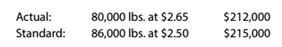Chapter 13, Problem 13.20E

Chapter
Section
Textbook Problem

Direct materials variancesThe following data relate to the direct materials cost for the production of 20,000 automobile tires:a.Determine the price variance, quantity variance, and total direct materials cost variance. b. To whom should the variances be reported for analysis and control?

To determine

Concept Introduction:

Direct material variances:

Direct material variances refer to the difference between the standard direct material cost and actual direct material cost incurred. Direct material cost variances are categorized into following two categories:

1. Direct material Rate variance: this variance shows the difference of standard rate and actual rate of material. The formula to calculate this variance is as follows:
2.   Direct material rate variance = (Actual rate – Standard rate) ×Actual Quantity

3. Direct material usage variance: this variance shows the difference of standard usage and actual usage of material. The formula to calculate this variance is as follows:
4.   Direct material usage variance = (Actual Quantity – Standard Quantity)×Standard rate

5. Direct material cost variance: this variance shows the difference of standard cost and actual cost of material. The formula to calculate this variance is as follows:
6.   Direct material cost variance = Direct material rate variance + Direct material usage variance

Requirement-1:

To Calculate:

The Direct Material Price, Quantity and Cost variances

Explanation

The Direct Material Price, Quantity and Cost variances are calculated as follows:

 Direct Material Price Variance: Actual Price (A) $2.65 Per lbs Standard Price (B)$ 2.50 Per lbs Actual Quantity (C) 80000 Ounces Direct Material Price Variance (D)= (A-B)*C = \$ 12,000.00 Unfavorable
To determine

Concept Introduction:

Direct material variances:

Direct material variances refer to the difference between the standard direct material cost and actual direct material cost incurred. Direct material cost variances are categorized into following two categories:

1. Direct material Rate variance: this variance shows the difference of standard rate and actual rate of material. The formula to calculate this variance is as follows:
2.   Direct material rate variance = (Actual rate – Standard rate) ×Actual Quantity

3. Direct material usage variance: this variance shows the difference of standard usage and actual usage of material. The formula to calculate this variance is as follows:
4.   Direct material usage variance = (Actual Quantity – Standard Quantity)×Standard rate

5. Direct material cost variance: this variance shows the difference of standard cost and actual cost of material. The formula to calculate this variance is as follows:
6.   Direct material cost variance = Direct material rate variance + Direct material usage variance

Requirement-2:

To Indicate:

The Person to whom the variances should be reported

Still sussing out bartleby?

Check out a sample textbook solution.

See a sample solution

The Solution to Your Study Problems

Bartleby provides explanations to thousands of textbook problems written by our experts, many with advanced degrees!

Get Started

Identify four disadvantages of a partnership form of business organization.

College Accounting, Chapters 1-27 (New in Accounting from Heintz and Parry)

EFFECTIVE INTEREST RATE You borrow 85,000; the annual loan payments are 8,273.59 for 30 years. What interest ra...

Fundamentals of Financial Management, Concise Edition (with Thomson ONE - Business School Edition, 1 term (6 months) Printed Access Card) (MindTap Course List)

What are the disadvantages of a corporation?

Foundations of Business (MindTap Course List)

Describe backup and recovery.

Accounting Information Systems

What is a predetermined overhead rate? Explain why it is used.

Cornerstones of Cost Management (Cornerstones Series)